Next: About this document ... Up: lab_template Previous: lab_template

Subsections

# Sequences and Series

## Introduction

The purpose of this lab is to learn how to define sequences and series using Maple as well as observe their plots and test for convergence.

## Getting Started

To assist you, there is a worksheet associated with this lab that contains examples similar to some of the exercises. You can copy that worksheet to your home directory with the following command, which must be run in a terminal window, not in Maple.
cp /math/calclab/MA1023/Seq_Series_start.mws ~/My_Documents

You can copy the worksheet now, but you should read through the lab before you load it into Maple. Once you have read through the exercises, start up Maple, load the worksheet, and go through it carefully. Then you can start working on the exercises.

## Background

An infinite series is the sum of an infinite sequence. More precisely, the sum of an infinite series is defined as the limit of the sequence of the partial sums of the terms of the series, provided this limit exists. If no finite limit exists, then we say that the series is divergent. The sum of an infinite series is defined as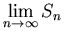, where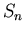is the partial sum of the first n terms of the series. However, because of the algebraic difficulty of expressingas a function of n, it is usually not possible to find sums by directly using the definition. So, if we can generally not work from the definition, what can be done? There are several convergence tests that provide us with some needed tools. These are tests that tell us if a series converges, but in the case that the series does converge, does not tell us the sum of the series. The convergence tests that will be used in this lab are the integral test and the ratio test.

## The Integral Test

The Integral Test for convergence is a method used to test convergence of an infinite series of nonnegative terms. The series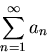converges if and only if the integral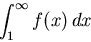is finite, whereis a positive, non-increasing and continuous function defined on the interval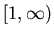and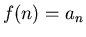an for all.

## The Ratio Test

The Ratio Test for convergence of a series can be thought of as a measurement of how fast the series is increasing or decreasing. This can be found by looking at the ratio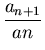as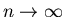. Given the series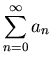, suppose that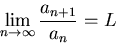Then
1. the series converges if,
2. the series diverges if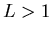,
3. the test is inconclusive if.

## Exercises

1. Write the sequence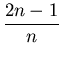as a function.
2. Plot the first fifteen terms.
3. Does it appear to converge or diverge? If it seems to converge, to what does it converge?
4. Write a function for the sum of the sequence.
5. Plot the sequence of partial sums.
6. Does it appear that the series converges or diverges? If it seems to converge, to what does it converge?
1. Apply the integral test to determine if the given series converges.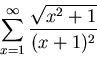1. First set up the function.
2. Plot the function. Does it seem to satisfy the necesary conditions?
3. Take the limit of the integral. What can you conclude and why?
2. Apply the ratio test to the given series.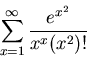1. First set up the function.
2. Take the limit of the ratio. What do you conclude and why?Next: About this document ... Up: lab_template Previous: lab_template
Dina Solitro
2006-02-02